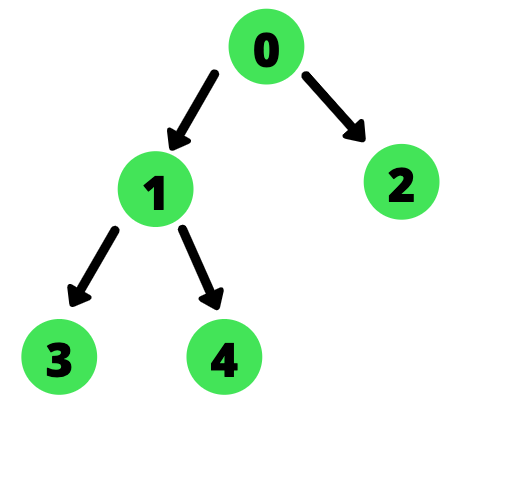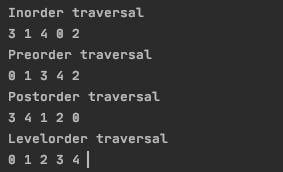# Breadth-First Search (BFS) and Depth-First Search (DFS) for Binary Trees in Java

Published on August 3, 2022By Jayant VermaWhile we believe that this content benefits our community, we have not yet thoroughly reviewed it. If you have any suggestions for improvements, please let us know by clicking the “report an issue“ button at the bottom of the tutorial.

Breadth-First Search and Depth-First Search are two techniques of traversing graphs and trees. In this tutorial, we will focus mainly on BFS and DFS traversals in trees.

## What is Depth First Search (DFS)?

The algorithm begins at the root node and then it explores each branch before backtracking. It is implemented using stacks. Often while writing the code, we use recursion stacks to backtrack. By using recursion we are able to take advantage of the fact that left and right subtrees are also trees and share the same properties.

For Binary trees, there are three types of DFS traversals.

1. In-Order
2. Pre-Order
3. Post-Order

## What is Breadth-First Search (BFS)?

This algorithm also begins at the root node and then visits all nodes level by level. That means after the root, it traverses all the direct children of the root. After all direct children of the root are traversed, it moves to their children and so on. To implement BFS we use a queue.

For Binary trees, we have Level Order Traversal which follows BFS.

## Implementation of BFS and DFS in Java

Let the tree under consideration be:The structure of TreeNode class is as follows :

`````` static class TreeNode {
int data;
TreeNode left, right;

public TreeNode(int key) {
data = key;
left = right = null;
}
}
``````

### 1. Pre-Order Traversal

In pre-order traversal of a binary tree, we first traverse the root, then the left subtree and then finally the right subtree. We do this recursively to benefit from the fact that left and right subtrees are also trees.

The algorithm for pre-order traversal is as follows:

1. Traverse the root.
2. Call preorder() on the left subtree.
3. Call preorder() on the right subtree.

The Pre-Order traversal of the tree above is:

``````0 1 3 4 2
``````

The java code is as follows:

`````` static void preorder(TreeNode TreeNode) {
if (TreeNode == null)
return;

// Traverse root
System.out.print(TreeNode.item + "->");
// Traverse left
preorder(TreeNode.left);
// Traverse right
preorder(TreeNode.right);
}
``````

### 2. In-Order Traversal

In-order traversal of a binary tree first traverses the left subtree then the root and finally the right subtree.

The algorithm for in-order traversal is as follows:

1. Call inorder() on left subtree.
2. Traverse the root.
3. Call inorder() on right subtree.

The in-order traversal of the tree above is:

``````3 1 4 0 2
``````

The java code is as follows :

`````` static void inorder(TreeNode TreeNode) {
if (TreeNode == null)
return;

// Traverse left
inorder(TreeNode.left);
// Traverse root
System.out.print(TreeNode.item + "->");
// Traverse right
inorder(TreeNode.right);
}
``````

### 3. Post-Order Traversal

Post-order traversal of a binary tree first traverses the left subtree then the right subtree and then finally the root.

The algorithm for post-order traversal is as follows:

1. Call postorder() on left subtree.
2. Call postorder() on right subtree.
3. Traverse the root.

The post-order traversal of the tree above is:

``````3 4 1 2 0
``````

The java code is as follows :

`````` static void postorder(TreeNode TreeNode) {
if (TreeNode == null)
return;

// Traverse left
postorder(TreeNode.left);
// Traverse right
postorder(TreeNode.right);
// Traverse root
System.out.print(TreeNode.item + "->");
}
``````

### 4. Level-Order Traversal

Level order traversal uses a queue to keep track of nodes to visit. After visiting a node, its children are put in the queue. To get a new node to traverse, we take out elements from the queue.

The algorithm is as follows:

1. Initialize an empty queue
3. Run a Loop till queue is not empty
1. Print data from temp.
2. Enqueue temp’s children in the order left then right.
3. Dequeue a node from the queue and assign it’s value to temp.

Level order traversal of the tree above is :

``````0 1 2 3 4
``````

The java code is as follows :

`````` static void printLevelOrder(TreeNode root) {
while (!queue.isEmpty()) {
TreeNode temp = queue.poll();
System.out.print(temp.data + " ");

/*add left child to the queue */
if (temp.left != null) {
}

/*add right right child to the queue */
if (temp.right != null) {
}
}
}
``````

## Complete Code Implementation of BFS and DFS in Java

The complete Java code is given below :

``````package com.JournalDev;
import java.util.Queue;

public class Main {
static class TreeNode {
int data;
TreeNode left, right;

public TreeNode(int key) {
data = key;
left = right = null;
}
}

static void preorder(TreeNode TreeNode) {
if (TreeNode == null)
return;

// Traverse root
System.out.print(TreeNode.data + " ");
// Traverse left
preorder(TreeNode.left);
// Traverse right
preorder(TreeNode.right);
}

static void inorder(TreeNode TreeNode) {
if (TreeNode == null)
return;

// Traverse left
inorder(TreeNode.left);
// Traverse root
System.out.print(TreeNode.data + " ");
// Traverse right
inorder(TreeNode.right);
}

static void postorder(TreeNode TreeNode) {
if (TreeNode == null)
return;

// Traverse left
postorder(TreeNode.left);
// Traverse right
postorder(TreeNode.right);
// Traverse root
System.out.print(TreeNode.data + " ");
}
static void printLevelOrder(TreeNode root) {
while (!queue.isEmpty()) {
TreeNode tempNode = queue.poll();
System.out.print(tempNode.data + " ");

/*add left child to the queue */
if (tempNode.left != null) {
}

/*add right right child to the queue */
if (tempNode.right != null) {
}
}
}

public static void main(String args[])

{
TreeNode root = new TreeNode(0);
root.left = new TreeNode(1);
root.right = new TreeNode(2);
root.left.left = new TreeNode(3);
root.left.right = new TreeNode(4);
System.out.println("Inorder traversal");
inorder(root);

System.out.println("\nPreorder traversal ");
preorder(root);

System.out.println("\nPostorder traversal");
postorder(root);

System.out.println("\nLevelorder traversal");
printLevelOrder(root);

}

}

``````
``````Output :

Inorder traversal
3 1 4 0 2
Preorder traversal
0 1 3 4 2
Postorder traversal
3 4 1 2 0
Levelorder traversal
0 1 2 3 4

``````## Conclusion

This tutorial was about BFS and DFS traversals in binary trees. To get DFS implementation in C++ refer to this tutorial. For C++ implementation of level order traversal refer to this tutorial.

Thanks for learning with the DigitalOcean Community. Check out our offerings for compute, storage, networking, and managed databases.Jayant Verma

author

#### Still looking for an answer?

Ask a questionSearch for more help

JournalDev
DigitalOcean EmployeeAugust 27, 2021

where is the code for DFS?

- jd

JournalDev
DigitalOcean EmployeeAugust 18, 2021

How do i know which part is bfs or dfs

- Raj

Click below to sign up and get \$200 of credit to try our products over 60 days!

### Popular Topics##### Hollie's Hub for Good

Working on improving health and education, reducing inequality, and spurring economic growth? We’d like to help.##### Become a contributor

You get paid; we donate to tech nonprofits.

##### Welcome to the developer cloud

DigitalOcean makes it simple to launch in the cloud and scale up as you grow – whether you’re running one virtual machine or ten thousand.© 2023 DigitalOcean, LLC.